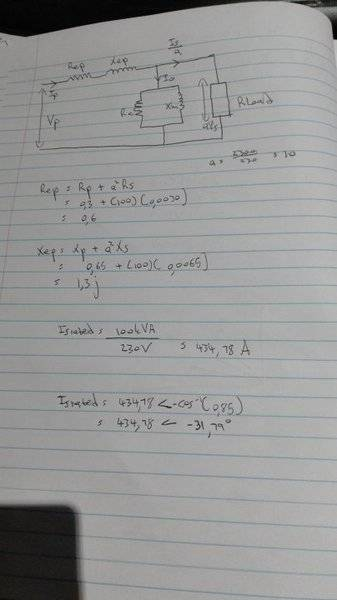# Single phase transformer question

## Homework Statement

A 100 kVA, 2300/230V, single phase transformer has the following parameters: Rp = 0.30Ω, Rs = 0.0030Ω, Rc = 4.5 kΩ, Xp = 0.65Ω, Xs = 0.0065Ω, Xm = 1.0 kΩ The transformer delivers 75 kW at 230 V at 0.85 power factor lagging, find:
a) The input current.
b) The input voltage.

## The Attempt at a Solution

[/B]
Referred to primaryI'm confused with the part where the transformer delivers 75kW. Then will the secondary current be 0.75*Israted ?

NascentOxygen
Staff Emeritus
I'm confused with the part where the transformer delivers 75kW. Then will the secondary current be 0.75*Israted ?
You know the power and the PF so you can determine the secondary's VA output.

You know the power and the PF so you can determine the secondary's VA output.

So,
cos(∅) = P/S
S = P/cos(∅)
= 75/0.85
= 88.24 kVA

So would the secondary current be
Is = 88.24/230 = 383.65A ?

NascentOxygen
Staff Emeritus
So,
cos(∅) = P/S
S = P/cos(∅)
= 75/0.85
= 88.24 kVA

So would the secondary current be
Is = 88.24/230 = 383.65A ?
That should be right.

Ok, so to find the input current, Ip, would this be right ?

From my schematic:

Ip = Io + Is/a..................1

Io = 2300(1/4.5k + 1/1k)

Is/a = 383.65∠-31.79 / 10
= 38.365∠-31.79

Therefore,

Ip = 2300(1/4.5k + 1/1kj) + 38.365∠-31.79
= 40.05∠-34.2

NascentOxygen
Staff Emeritus
Ok, so to find the input current, Ip, would this be right ?

From my schematic:

Ip = Io + Is/a..................1

Io = 2300(1/4.5k + 1/1k)

Is/a = 383.65∠-31.79 / 10
= 38.365∠-31.79

Therefore,

Ip = 2300(1/4.5k + 1/1kj) + 38.365∠-31.79
= 40.05∠-34.2
That looks right.

That looks right.
Thanks